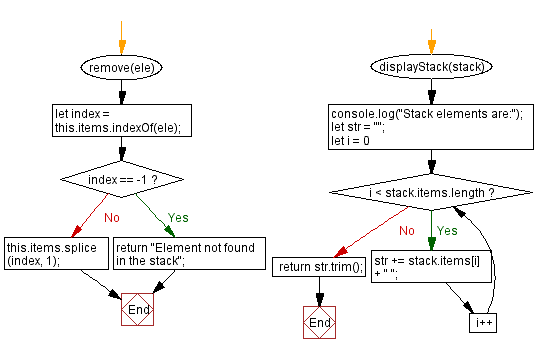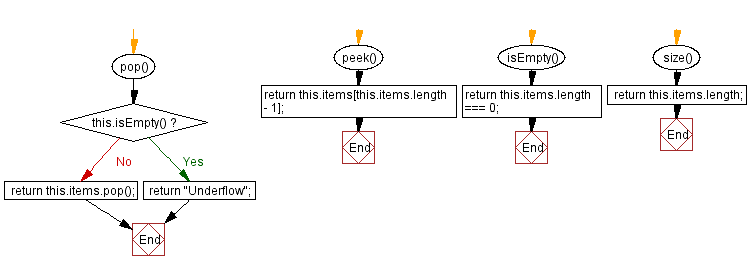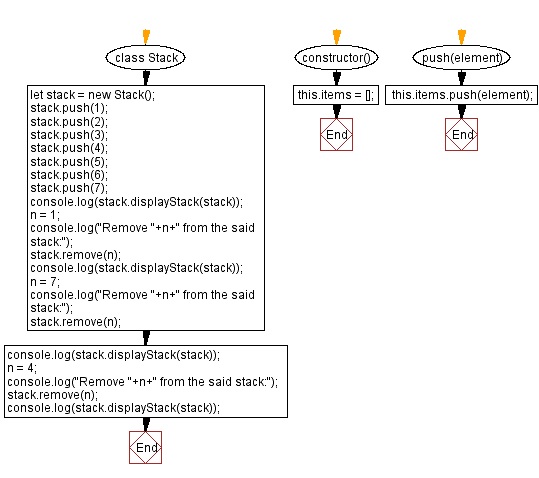# JavaScript Exercises: Remove a specific element from a stack

## JavaScript Stack: Exercise-14 with Solution

Write a JavaScript program to remove a specific element from a stack.

Sample Solution:

JavaScript Code:

``````class Stack {
constructor() {
this.items = [];
}
push(element) {
this.items.push(element);
}
pop() {
if (this.isEmpty()) {
return "Underflow";
}
return this.items.pop();
}
peek() {
return this.items[this.items.length - 1];
}
isEmpty() {
return this.items.length === 0;
}
size() {
return this.items.length;
}
remove(ele) {
let index = this.items.indexOf(ele);
if (index == -1) {
}
this.items.splice(index, 1);
return;
}
displayStack(stack) {
console.log("Stack elements are:");
let str = "";
for (let i = 0; i < stack.items.length; i++)
str += stack.items[i] + " ";
return str.trim();
}
}
let stack = new Stack();
stack.push(1);
stack.push(2);
stack.push(3);
stack.push(4);
stack.push(5);
stack.push(6);
stack.push(7);
console.log(stack.displayStack(stack));
n = 1;
console.log("Remove "+n+" from the said stack:");
stack.remove(n);
console.log(stack.displayStack(stack));
n = 7;
console.log("Remove "+n+" from the said stack:");
stack.remove(n);
console.log(stack.displayStack(stack));
n = 4;
console.log("Remove "+n+" from the said stack:");
stack.remove(n);
console.log(stack.displayStack(stack));
```
```

Sample Output:

```Stack elements are:
1 2 3 4 5 6 7
Remove 1 from the said stack:
Stack elements are:
2 3 4 5 6 7
Remove 7 from the said stack:
Stack elements are:
2 3 4 5 6
Remove 4 from the said stack:
Stack elements are:
2 3 5 6
```

Flowchart:Live Demo:

See the Pen javascript-stack-exercise-14 by w3resource (@w3resource) on CodePen.

Improve this sample solution and post your code through Disqus

Stack Previous: Middle element(s) of a stack.
Stack Exercises Next: Swap the top two elements of a stack.

What is the difficulty level of this exercise?

Test your Programming skills with w3resource's quiz.

﻿